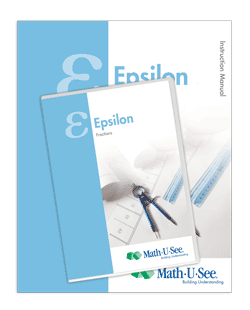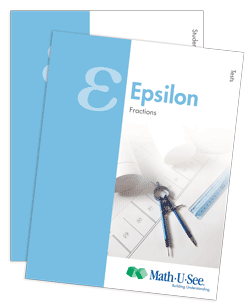# Epsilon## Updated Epsilon — Available March 2013

Fractions and Other Topics

Now that students have learned basic operations with whole numbers, Epsilon covers these same operations with fractions. Fractions are presented in an intuitive way with visual explanations of equivalent fractions, common denominators, and fractions and numbers larger than 1. Fractions and operations are illustrated using our proprietary Fraction Overlay manipulatives.Updates to Instruction Manual include clarification and definition of:

• decomposition
• unit fraction
• rectangular prism
• other concepts previously taught or impliedNew Application & Enrichment pages in the Student Workbook include:

• focus on a variety of approaches to understand
fraction word problems and operations of fractions
• using parentheses and brackets to rewrite problems (distributive property)
• classifying polygons
• building volume problems
• patterns
• using a chart to convert measurement units
• graphing coordinate points (positive only)

Major Concepts and Skills Include:

• Recognizing and generating equivalent fractions
• Understanding addition, subtraction, multiplication, and division of fractions and mixed numbers
• Fluently adding, subtracting, multiplying, and dividing fractions and mixed numbers

• Using multiple strategies to recognize common factors
• Understanding grouping symbols and their effect on order of operations
• Interpreting and solving word problems
• Comparing, and converting decimal fractions
• Finding the area and circumference of circles
• Representing fractions and fractional measurements on line plots and number lines
• Using coordinates to represent ordered relationships

Please check back in the first week of March for ordering information.

Live Chat

9 AM to 6 PM Eastern time,
Monday through Friday

Phone Support

Call (888) 854-6284
International callers —
call +1 717 283 1448

8:30 AM to 6 PM Eastern time,
Monday through Friday

Email